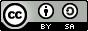# 个性化推荐¶

## 说明:¶

1. 硬件环境要求： 本文可支持在CPU、GPU下运行
2. Docker镜像支持的CUDA/cuDNN版本： 如果使用了Docker运行Book，请注意：这里所提供的默认镜像的GPU环境为 CUDA 8/cuDNN 5，对于NVIDIA Tesla V100等要求CUDA 9的 GPU，使用该镜像可能会运行失败。
3. 文档和脚本中代码的一致性问题： 请注意：为使本文更加易读易用，我们拆分、调整了train.py的代码并放入本文。本文中代码与train.py的运行结果一致，可直接运行train.py进行验证。

## 背景介绍¶

1994年明尼苏达大学推出的GroupLens系统一般被认为是个性化推荐系统成为一个相对独立的研究方向的标志。该系统首次提出了基于协同过滤来完成推荐任务的思想，此后，基于该模型的协同过滤推荐引领了个性化推荐系统十几年的发展方向。

• 协同过滤推荐（Collaborative Filtering Recommendation）：该方法是应用最广泛的技术之一，需要收集和分析用户的历史行为、活动和偏好。它通常可以分为两个子类：基于用户 （User-Based）的推荐 和基于物品（Item-Based）的推荐。该方法的一个关键优势是它不依赖于机器去分析物品的内容特征，因此它无需理解物品本身也能够准确地推荐诸如电影之类的复杂物品；缺点是对于没有任何行为的新用户存在冷启动的问题，同时也存在用户与商品之间的交互数据不够多造成的稀疏问题。值得一提的是，社交网络或地理位置等上下文信息都可以结合到协同过滤中去。
• 基于内容过滤推荐（Content-based Filtering Recommendation）：该方法利用商品的内容描述，抽象出有意义的特征，通过计算用户的兴趣和商品描述之间的相似度，来给用户做推荐。优点是简单直接，不需要依据其他用户对商品的评价，而是通过商品属性进行商品相似度度量，从而推荐给用户所感兴趣商品的相似商品；缺点是对于没有任何行为的新用户同样存在冷启动的问题。
• 组合推荐（Hybrid Recommendation）：运用不同的输入和技术共同进行推荐，以弥补各自推荐技术的缺点。

## 效果展示¶

Input movie_id: 1962
Input user_id: 1
Prediction Score is 4.25


## 模型概览¶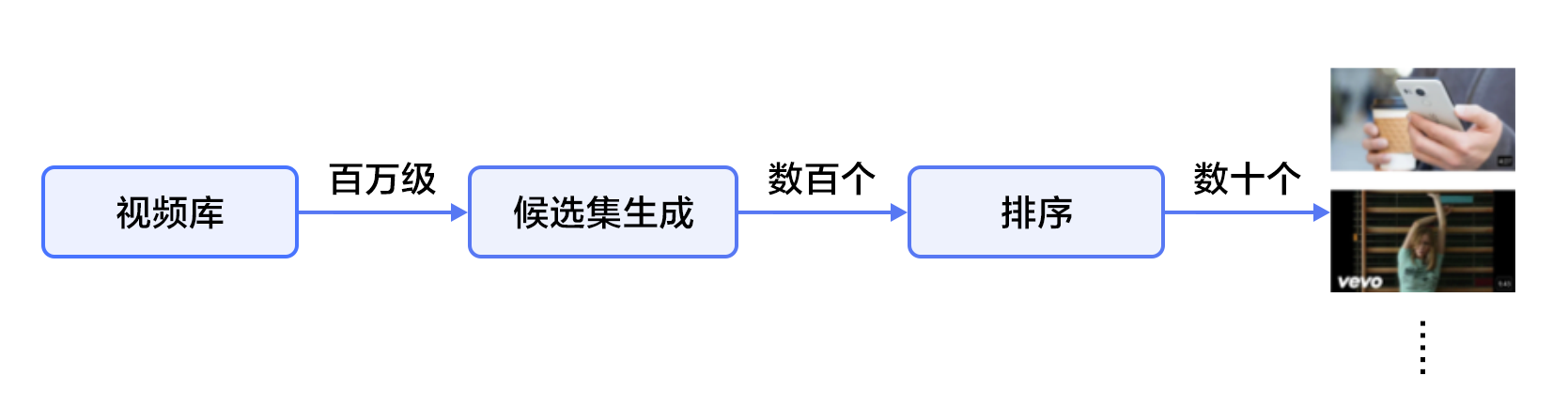#### 候选生成网络（Candidate Generation Network）¶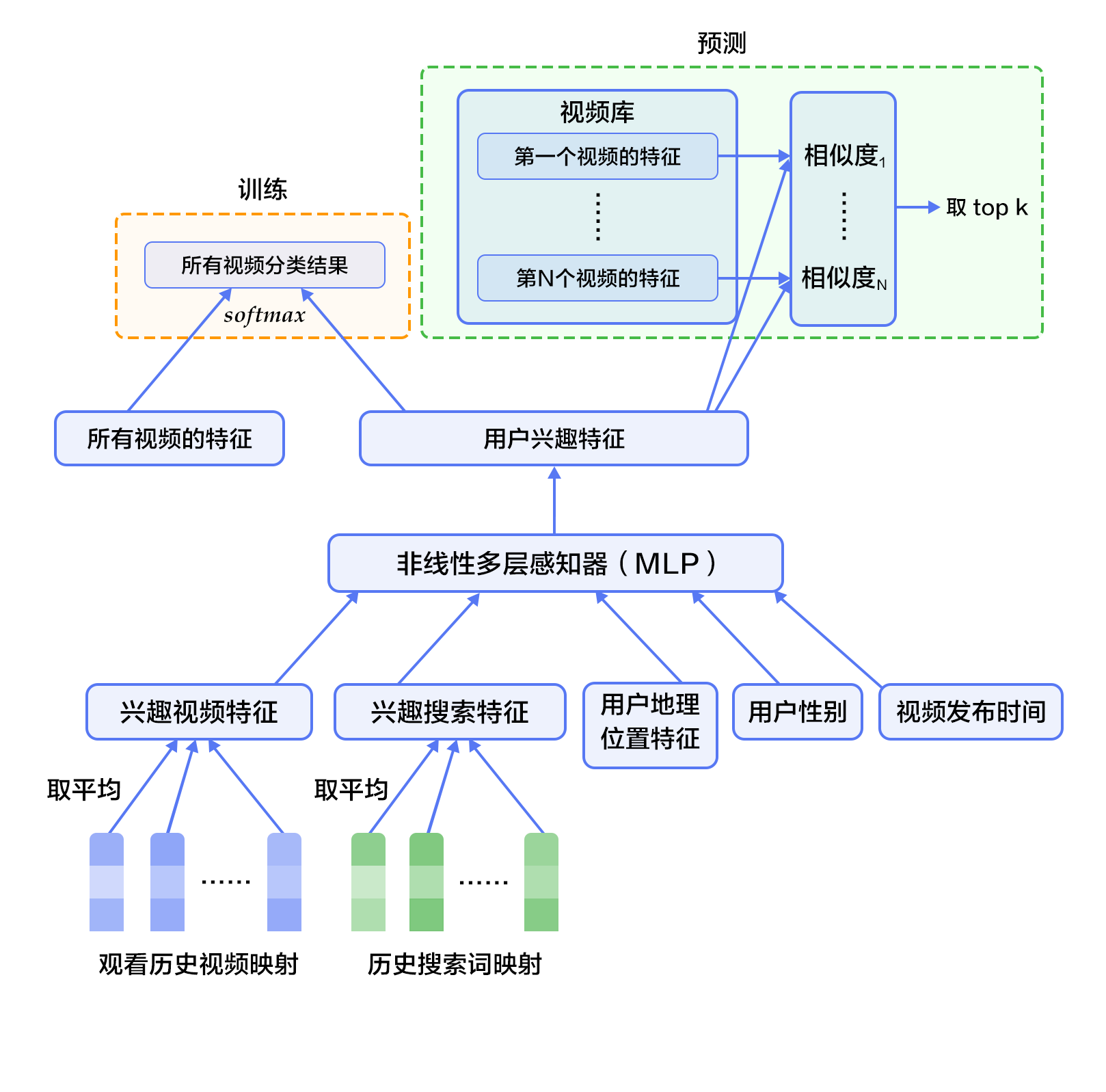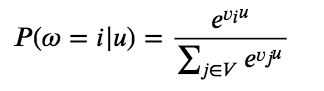### 融合推荐模型¶

#### 文本卷积神经网络（CNN）¶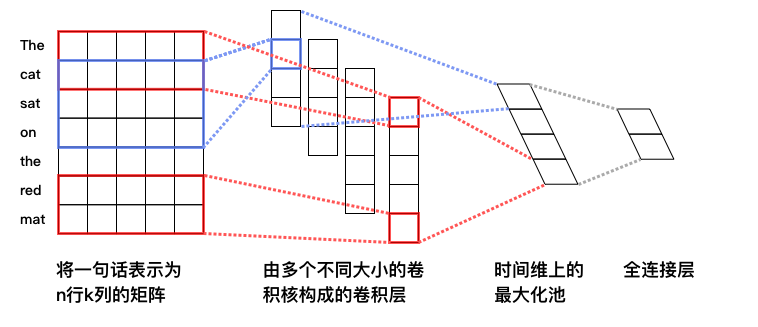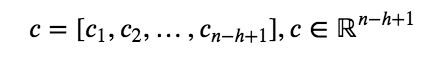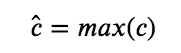#### 融合推荐模型概览¶

1. 首先，使用用户特征和电影特征作为神经网络的输入，其中：
• 用户特征融合了四个属性信息，分别是用户ID、性别、职业和年龄。
• 电影特征融合了三个属性信息，分别是电影ID、电影类型ID和电影名称。
2. 对用户特征，将用户ID映射为维度大小为256的向量表示，输入全连接层，并对其他三个属性也做类似的处理。然后将四个属性的特征表示分别全连接并相加。
3. 对电影特征，将电影ID以类似用户ID的方式进行处理，电影类型ID以向量的形式直接输入全连接层，电影名称用文本卷积神经网络得到其定长向量表示。然后将三个属性的特征表示分别全连接并相加。
4. 得到用户和电影的向量表示后，计算二者的余弦相似度作为个性化推荐系统的打分。最后，用该相似度打分和用户真实打分的差异的平方作为该回归模型的损失函数。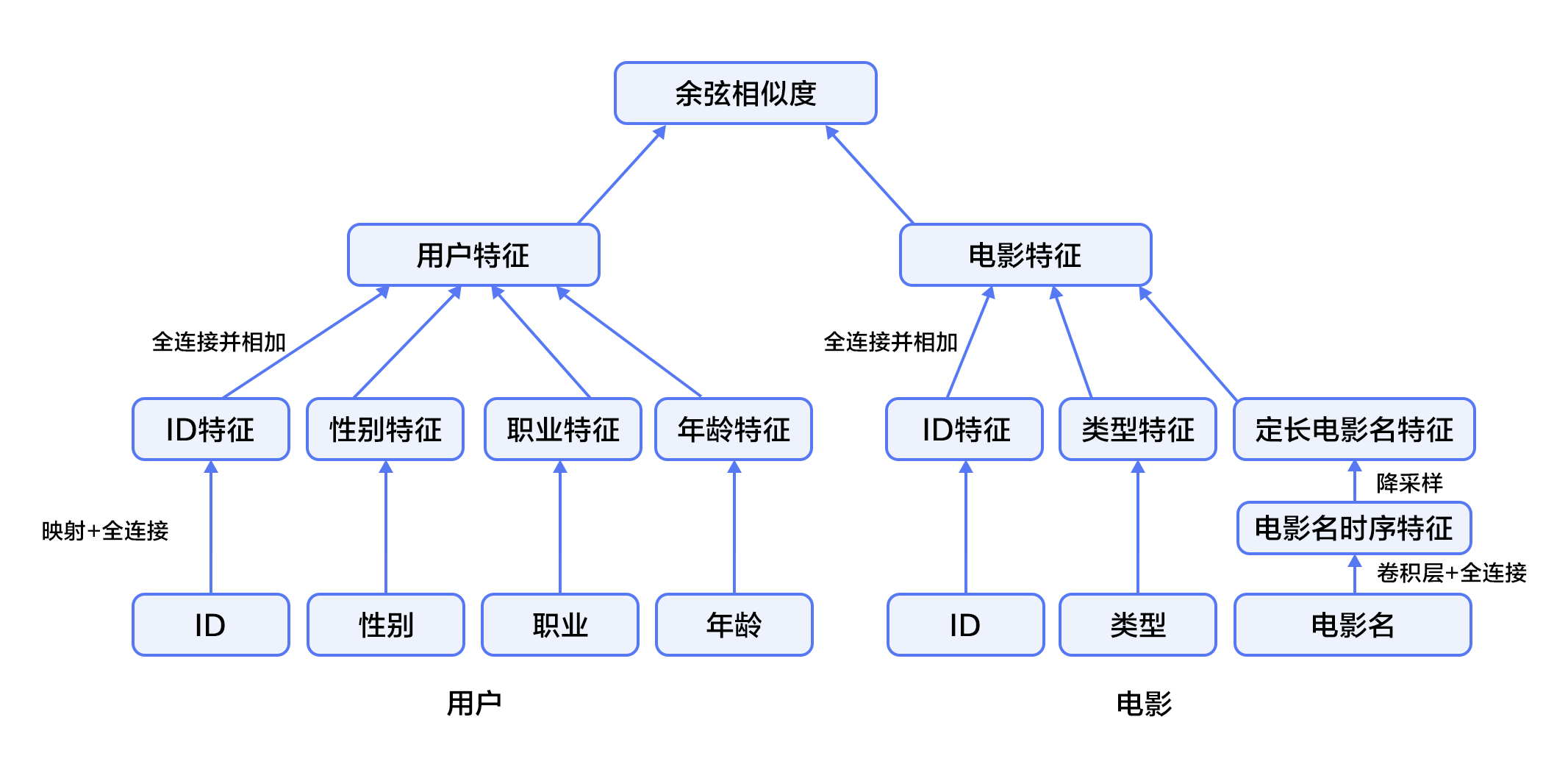## 数据准备¶

### 数据介绍与下载¶

Paddle在API中提供了自动加载数据的模块。数据模块为 paddle.dataset.movielens

from __future__ import print_function
print(list(movie_info.values()))

# Run this block to show dataset's documentation


movie_info = paddle.dataset.movielens.movie_info()
print(list(movie_info.values()))

<MovieInfo id(1), title(Toy Story ), categories(['Animation', "Children's", 'Comedy'])>


user_info = paddle.dataset.movielens.user_info()
print(list(user_info.values()))

<UserInfo id(1), gender(F), age(1), job(10)>


• 1: "Under 18"
• 18: "18-24"
• 25: "25-34"
• 35: "35-44"
• 45: "45-49"
• 50: "50-55"
• 56: "56+"

• 0: "other" or not specified
• 2: "artist"
• 5: "customer service"
• 6: "doctor/health care"
• 7: "executive/managerial"
• 8: "farmer"
• 9: "homemaker"
• 10: "K-12 student"
• 11: "lawyer"
• 12: "programmer"
• 13: "retired"
• 14: "sales/marketing"
• 15: "scientist"
• 16: "self-employed"
• 17: "technician/engineer"
• 19: "unemployed"
• 20: "writer"

train_set_creator = paddle.dataset.movielens.train()
train_sample = next(train_set_creator())
uid = train_sample
mov_id = train_sample[len(user_info[uid].value())]
print ("User %s rates Movie %s with Score %s"%(user_info[uid], movie_info[mov_id], train_sample[-1]))

User <UserInfo id(1), gender(F), age(1), job(10)> rates Movie <MovieInfo id(1193), title(One Flew Over the Cuckoo's Nest ), categories(['Drama'])> with Score [5.0]


## 模型配置说明¶

• IS_SPARSE: embedding中是否使用稀疏更新
• PASS_NUM: epoch数量
import math
import sys
import numpy as np

IS_SPARSE = True
BATCH_SIZE = 256
PASS_NUM = 20


def get_usr_combined_features():
"""network definition for user part"""

uid = fluid.data(name='user_id', shape=[None], dtype='int64')

usr_emb = fluid.embedding(
input=uid,
dtype='float32',
size=[USR_DICT_SIZE, 32],
param_attr='user_table',
is_sparse=IS_SPARSE)

usr_fc = layers.fc(input=usr_emb, size=32)

USR_GENDER_DICT_SIZE = 2

usr_gender_id = fluid.data(name='gender_id', shape=[None], dtype='int64')

usr_gender_emb = fluid.embedding(
input=usr_gender_id,
size=[USR_GENDER_DICT_SIZE, 16],
param_attr='gender_table',
is_sparse=IS_SPARSE)

usr_gender_fc = layers.fc(input=usr_gender_emb, size=16)

usr_age_id = fluid.data(name='age_id', shape=[None], dtype="int64")

usr_age_emb = fluid.embedding(
input=usr_age_id,
size=[USR_AGE_DICT_SIZE, 16],
is_sparse=IS_SPARSE,
param_attr='age_table')

usr_age_fc = layers.fc(input=usr_age_emb, size=16)

usr_job_id = fluid.data(name='job_id', shape=[None], dtype="int64")

usr_job_emb = fluid.embedding(
input=usr_job_id,
size=[USR_JOB_DICT_SIZE, 16],
param_attr='job_table',
is_sparse=IS_SPARSE)

usr_job_fc = layers.fc(input=usr_job_emb, size=16)

concat_embed = layers.concat(
input=[usr_fc, usr_gender_fc, usr_age_fc, usr_job_fc], axis=1)

usr_combined_features = layers.fc(input=concat_embed, size=200, act="tanh")

return usr_combined_features


def get_mov_combined_features():
"""network definition for item(movie) part"""

mov_id = fluid.data(name='movie_id', shape=[None], dtype='int64')

mov_emb = fluid.embedding(
input=mov_id,
dtype='float32',
size=[MOV_DICT_SIZE, 32],
param_attr='movie_table',
is_sparse=IS_SPARSE)

mov_fc = layers.fc(input=mov_emb, size=32)

category_id = fluid.data(
name='category_id', shape=[None], dtype='int64', lod_level=1)

mov_categories_emb = fluid.embedding(
input=category_id, size=[CATEGORY_DICT_SIZE, 32], is_sparse=IS_SPARSE)

mov_categories_hidden = layers.sequence_pool(
input=mov_categories_emb, pool_type="sum")

mov_title_id = fluid.data(
name='movie_title', shape=[None], dtype='int64', lod_level=1)

mov_title_emb = fluid.embedding(
input=mov_title_id, size=[MOV_TITLE_DICT_SIZE, 32], is_sparse=IS_SPARSE)

mov_title_conv = nets.sequence_conv_pool(
input=mov_title_emb,
num_filters=32,
filter_size=3,
act="tanh",
pool_type="sum")

concat_embed = layers.concat(
input=[mov_fc, mov_categories_hidden, mov_title_conv], axis=1)

mov_combined_features = layers.fc(input=concat_embed, size=200, act="tanh")

return mov_combined_features


def inference_program():
"""the combined network"""

usr_combined_features = get_usr_combined_features()
mov_combined_features = get_mov_combined_features()

inference = layers.cos_sim(X=usr_combined_features, Y=mov_combined_features)
scale_infer = layers.scale(x=inference, scale=5.0)

return scale_infer


def train_program():
"""define the cost function"""

scale_infer = inference_program()

label = fluid.data(name='score', shape=[None, 1], dtype='float32')
square_cost = layers.square_error_cost(input=scale_infer, label=label)
avg_cost = layers.mean(square_cost)

return [avg_cost, scale_infer]

def optimizer_func():
return fluid.optimizer.SGD(learning_rate=0.2)


## 训练模型¶

### 定义训练环境¶

use_cuda = False
place = fluid.CUDAPlace(0) if use_cuda else fluid.CPUPlace()


### 定义数据提供器¶

train_reader = fluid.io.batch(
fluid.io.shuffle(
batch_size=BATCH_SIZE)



### 提供数据¶

feed_order用来定义每条产生的数据和paddle.layer.data之间的映射关系。比如，movielens.train产生的第一列的数据对应的是user_id这个特征。

feed_order = [
'user_id', 'gender_id', 'age_id', 'job_id', 'movie_id', 'category_id',
'movie_title', 'score'
]


### 构建训练程序以及测试程序¶

main_program = fluid.default_main_program()
star_program = fluid.default_startup_program()
[avg_cost, scale_infer] = train_program()

test_program = main_program.clone(for_test=True)
sgd_optimizer = optimizer_func()
sgd_optimizer.minimize(avg_cost)
exe = fluid.Executor(place)

count = 0
feed_var_list = [
program.global_block().var(var_name) for var_name in feed_order
]
feeder_test = fluid.DataFeeder(
feed_list=feed_var_list, place=place)
test_exe = fluid.Executor(place)
accumulated = 0
avg_cost_np = test_exe.run(program=program,
feed=feeder_test.feed(test_data),
fetch_list=[avg_cost])
accumulated += avg_cost_np
count += 1
return accumulated / count


### 构建训练主循环并开始训练¶

# Specify the directory path to save the parameters
params_dirname = "recommender_system.inference.model"

train_prompt = "Train cost"
test_prompt = "Test cost"

plot_cost = Ploter(train_prompt, test_prompt)

def train_loop():
feed_list = [
main_program.global_block().var(var_name) for var_name in feed_order
]
feeder = fluid.DataFeeder(feed_list, place)
exe.run(star_program)

for pass_id in range(PASS_NUM):
# train a mini-batch
outs = exe.run(program=main_program,
feed=feeder.feed(data),
fetch_list=[avg_cost])
out = np.array(outs)

# get test avg_cost

plot_cost.append(train_prompt, batch_id, outs)
plot_cost.append(test_prompt, batch_id, test_avg_cost)
plot_cost.plot()

if batch_id == 20:
if params_dirname is not None:
fluid.io.save_inference_model(params_dirname, [
"user_id", "gender_id", "age_id", "job_id",
"movie_id", "category_id", "movie_title"
], [scale_infer], exe)
return
print('EpochID {0}, BatchID {1}, Test Loss {2:0.2}'.format(
pass_id + 1, batch_id + 1, float(test_avg_cost)))

if math.isnan(float(out)):
sys.exit("got NaN loss, training failed.")


train_loop()


## 应用模型¶

### 生成测试数据¶

infer_movie_id = 783
user_id = np.array().astype("int64").reshape(-1)
gender_id = np.array().astype("int64").reshape(-1)
age_id = np.array().astype("int64").reshape(-1)
job_id = np.array().astype("int64").reshape(-1)
movie_id = np.array().astype("int64").reshape(-1) # Hunchback of Notre Dame
category_id = fluid.create_lod_tensor(np.array([10, 8, 9], dtype='int64'), [], place) # Animation, Children's, Musical
movie_title = fluid.create_lod_tensor(np.array([1069, 4140, 2923, 710, 988], dtype='int64'), [],
place) # 'hunchback','of','notre','dame','the'


### 构建预测过程并测试¶

place = fluid.CUDAPlace(0) if use_cuda else fluid.CPUPlace()
exe = fluid.Executor(place)

inference_scope = fluid.core.Scope()


### 测试¶

with fluid.scope_guard(inference_scope):
[inferencer, feed_target_names,

results = exe.run(inferencer,
feed={
'user_id': user_id,
'gender_id': gender_id,
'age_id': age_id,
'job_id': job_id,
'movie_id': movie_id,
'category_id': category_id,
'movie_title': movie_title
},
fetch_list=fetch_targets,
return_numpy=False)
predict_rating = np.array(results)
print("Predict Rating of user id 1 on movie \"" + infer_movie_name +
"\" is " + str(predict_rating))
print("Actual Rating of user id 1 on movie \"" + infer_movie_name +
"\" is 4.")


## 参考文献¶

1. P. Resnick, N. Iacovou, etc. “GroupLens: An Open Architecture for Collaborative Filtering of Netnews”, Proceedings of ACM Conference on Computer Supported Cooperative Work, CSCW 1994. pp.175-186.
2. Sarwar, Badrul, et al. "Item-based collaborative filtering recommendation algorithms.Proceedings of the 10th international conference on World Wide Web. ACM, 2001.
3. Kautz, Henry, Bart Selman, and Mehul Shah. "Referral Web: combining social networks and collaborative filtering." Communications of the ACM 40.3 (1997): 63-65. APA
4. Peter Brusilovsky (2007). The Adaptive Web. p. 325.
5. Robin Burke , Hybrid Web Recommender Systems, pp. 377-408, The Adaptive Web, Peter Brusilovsky, Alfred Kobsa, Wolfgang Nejdl (Ed.), Lecture Notes in Computer Science, Springer-Verlag, Berlin, Germany, Lecture Notes in Computer Science, Vol. 4321, May 2007, 978-3-540-72078-2.
6. Yuan, Jianbo, et al. "Solving Cold-Start Problem in Large-scale Recommendation Engines: A Deep Learning Approach." arXiv preprint arXiv:1611.05480 (2016).
7. Covington P, Adams J, Sargin E. Deep neural networks for youtube recommendations[C]//Proceedings of the 10th ACM Conference on Recommender Systems. ACM, 2016: 191-198.